# Difference between revisions of "Compact times paracompact implies paracompact"

This article states and proves a result of the following form: the product of two topological spaces, the first satisfying the property Compact space (?) and the second satisfying the property Paracompact space (?), is a topological space satisfying the property Paracompact space (?).
View other such computations

## Statement

Let$X$ be a compact space and$Y$ a paracompact space. Then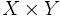$X \times Y$, the Cartesian product endowed with the product topology, is paracompact.

## Related facts

Other results using the same proof technique:

## Facts used

1. Tube lemma: Suppose$X$ is a compact space and$Y$ is a topological space. Then, given any open subset$U$ of$X \times Y$ containing$X \times \{ y \}$ for some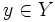$y \in Y$, there exists an open subset$V$ of$Y$ such that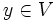$y \in V$ and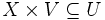$X \times V \subseteq U$.

## Proof

Given: A compact space$X$, a paracompact space$Y$.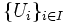$\{ U_i \}_{i \in I}$ form an open cover of$X \times Y$.

To prove: There exists a locally finite open refinement of the$U_i$s, i.e., an open cover$\{ Q_k \}_{k \in K}$ of$X \times Y$ such that:

• It is locally finite: For any point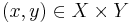$(x,y) \in X \times Y$, there exists an open set$R$ containing$(x,y)$ that intersects only finitely many of the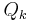$Q_k$s.
• It refines$\{ U_i \}_{i \in I}$: Every$Q_k$ is contained in one of the$U_i$s.

Proof: Note that in the proof below, Step (4) has three aspects (locally finite, cover, and refinement) and different later steps use different aspects of Step (4), with the specific aspect used indicated parenthetically in the Previous steps used column.

Step no. Assertion/construction Facts used Given data used Previous steps used Explanation
1 For any point$y \in Y$, there is a finite collection of$U_i$ that cover$X \times \{ y \}$.$X$ is compact Since$X$ is compact, the subspace$X \times \{ y \}$ of$X \times Y$ is also compact, so the cover by the open subsets$U_i$ has a finite subcover.
2 For any point$y \in Y$, let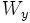$W_y$ be the union of the finite collection of open subsets$U_i$ as obtained in Step (1). There exists an open subset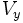$V_y$ of$Y$ such that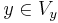$y \in V_y$ and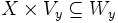$X \times V_y \subseteq W_y$. Fact (1)$X$ is compact Step (1) Follows from Fact (1), setting the$U$ of Fact (1) to be$W_y$.
3 The open subsets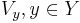$V_y, y \in Y$ obtained in Step (2) form an open cover of$Y$. Step (2) By Step (2),$y \in V_y$. Since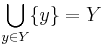$\bigcup_{y \in Y} \{ y \} = Y$, and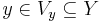$y \in V_y \subseteq Y$, we get$\bigcup_{y \in Y} V_y = Y$.
4 There exists a locally finite open refinement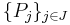$\{ P_j \}_{j \in J}$ of the$V_y$ in$Y$.$Y$ is paracompact Step (3) Step-given combination direct.
5 For each$P_j$,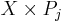$X \times P_j$ is a union of finitely many intersections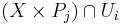$(X \times P_j) \cap U_i$, all of which are open subsets. Steps (1), (2), (4) (refinement aspect) [SHOW MORE]
6 The open subsets of the form$(X \times P_j) \cap U_i$ of Step (5) form an open cover of$X \times Y$ that refines the$U_i$s (note that not every combination of$P_j$ and$U_i$ is included -- only the finitely many$U_i$s needed as in Step (5)). We will index this open cover by indexing set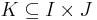$K \subseteq I \times J$, and call it$\{ Q_k \}_{k \in K}$, where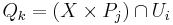$Q_k = (X \times P_j) \cap U_i$. In particular, if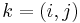$k = (i,j)$, then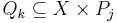$Q_k \subseteq X \times P_j$ and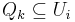$Q_k \subseteq U_i$, and for any$j$, there are finitely many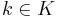$k \in K$ with the second coordinate of$k$ equal to$j$. Steps (4) (cover aspect), (5)$\{ P_j \}_{j \in J}$ cover$Y$, so$\{ X \times P_j \}_{j \in J}$ cover$X \times Y$. By Step (5),$X \times P_j$ is the union of finitely many$(X \times P_j) \cap U_i$, so the latter also form an open cover of$X \times Y$.
7 The open cover$\{ Q_k \}_{k \in K}$ of Step (6) is a locally finite open cover. In other words, for any$(x, y) \in X \times Y$, there is an open subset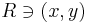$R \ni (x,y)$ such that$R$ intersects only finite many$Q_k$s. Steps (4) (locally finite aspect), (6) [SHOW MORE]
8 The open cover$\{ Q_k \}_{k \in K}$ is as desired Steps (6), (7) Combine the two steps to get what we wanted to prove.
This proof uses a tabular format for presentation. Learn more about tabular proof formats|View all pages on facts with proofs in tabular format